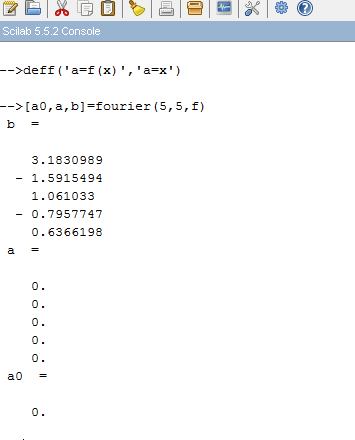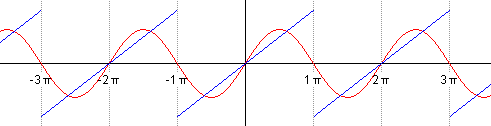# Fourier Series and SCILAB

Today I wrote a code that calculates the Fourier Coefficients.

In case you don’t know what a Fourier Series is, then, basically it is a way of approximating or representing a periodic function by a series of simple harmonic(sine and cosine) functions.

You can check out the wikipedia for  more information on it: https://en.wikipedia.org/wiki/Fourier_series

For a waveform
f(x) with period 2L=2π/k, we have that k=2π/2L=π/L and nkx =nπx/L , Then we have
So, here’s how my code works:
I created a function

[A0,A,B]=fourier(l,n,f)
Arguments:

l :half of the period, (periodicity of the function f which is to be approximated by Fourier Series)

n: no. of Fourier Coefficients you want to calculate

f: function which is to be approximated by Fourier Series

A0: The first fourier coefficient.

A: An array/matrix whose nth element is the nth coefficient An.

B: An array/matrix whose nth element is the nth coefficient Bn.

The code is:

```//Fourier Series Coefficients
//The following function returns the fourier coefficients,'a0', 'An' & 'Bn'
//
//User needs to provide the following arguments:
//
//l=periodicity of the function f which is to be approximated by Fourier Series
//n=no. of Fourier Coefficients you want to calculate
//f=function which is to be approximated by Fourier Series
//
//*Some necessary guidelines for defining f:
//*The program integrates the function f from -l to l so make sure you define the function f correctly in the interval -l to l.
//
//
//Written by: Manas Sharma([email protected])
//For more useful toolboxes and tutorials on Scilab visit: https://www.bragitoff.com/category/compu-geek/scilab/
funcprot(0);
function [a0, A, B]=fourier(l, n, f)
a0=1/l*intg(-l,l,f,1e-2);
for i=1:n
function b=f1(x, f)
b=f(x)*cos(i*%pi*x/l);
endfunction
function c=f2(x, f)
c=f(x)*sin(i*%pi*x/l);
endfunction
A(i)=1/l*intg(-l,l,f1,1e-2);
B(i)=1/l*intg(-l,l,f2,1e-2);
end
endfunction```

Output:Tutorial:

That’s it. I hope it was not too difficult to understand. If you have any questions I will be glad to answer them.[wpedon id="7041" align="center"]

## 10 thoughts on “Fourier Series and SCILAB”

1. Hi! thank you for the tutorial. I was just wondering if you need to change the function where would you have to make the change. For example if you have a square plot.

2. Hi,Thank you for the tutorial, I was wondering how to take fourier series of some other function for that matter of fact if i wanted to plot FS of triangular wave where and how would I define f(x) in this case.

1. Hi Lalith,
To find out the FS of any periodic function, you first need to understand how to define a periodic function. For that please check out this post: https://www.bragitoff.com/2016/03/defining-a-periodic-function-in-scilab/
Then you can also read another post on FS:
https://www.bragitoff.com/2016/03/calculating-fourier-series-and-plotting-it-scilab/
I have also created a toolbox for FS calculatoon and plotting. Links are in the post.
Good luck!

3. in exam one question came find Fourier series coefficient of f(x)=3x^2 in(-pi,pi)for n=5 .Plase reply how to solve this kind of problem

4. Hi,
Manas i need your help regarding a scilab prog.To solve the S -wave schrodinger equation for the ground state and first excited state of hydrogen atom :(m is the reduced mass of electron.Obtain the energy eigen value and plot the corresponding value wave function. e=3.795 (eVA)1/2, h=1973(eVA) and m=0.511*106 eV/c2

5. Hello Sir;
I want to just ask you that the error is displaying while executing this prog in my PC at line 20 i.e.
x=-5*l:0.1:5*l . Its showing that there is undefined variable ‘l’.
Can you just help me out.

1. I think you’re talking about the code posted here: https://www.bragitoff.com/2016/03/calculating-fourier-series-and-plotting-it-scilab/

That is the letter ‘L’ in lowercase, in case you were confusing it with capital ‘i’.

In case you copied it correctly, then I don’t understand why the error is there because ‘l’ is defined in the function definition:
function [a0,A,B]=fourierplot(l,n,f)

6. Madam i have a trignometric fourier series with all coefficients how can i plot it . do u have code forthat ?

7. Is this code for piecewise function too? i couldn’t use, so can you make the video for piecewise function too?